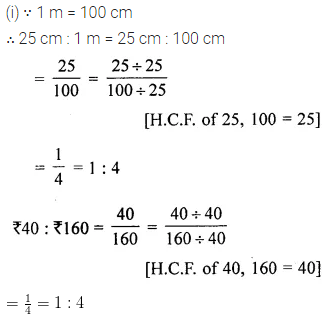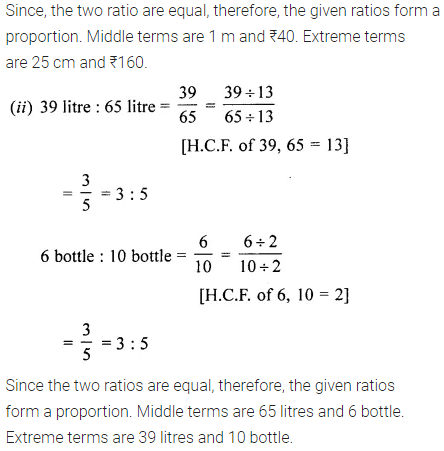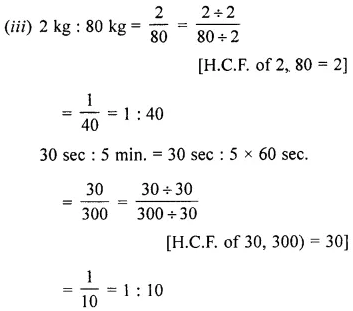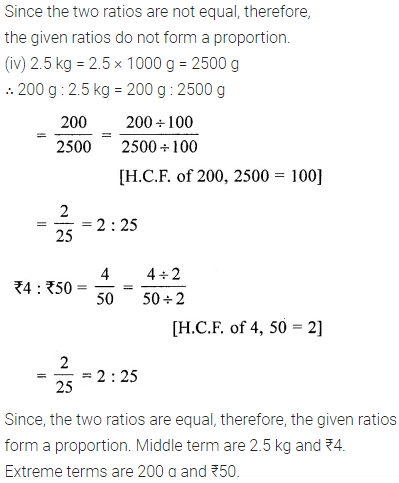# ML Aggarwal Class 6 Solutions for ICSE Maths Chapter 8 Ratio and Proportion Ex 8.2

## ML Aggarwal Class 6 Solutions for ICSE Maths Chapter 8 Ratio and Proportion Ex 8.2

Question 1.
Check whether the given two ratios form a proportion or not:
(i) 4 : 6 and 12 : 18
(ii) 15:45 and 40 :120
(iii) 14 : 4 and 18 : 6
(iv) 12 : 18 and 28 : 12
Solution:Question 2.
Write true (T) or false (F) against each of the following statements:
(i) 16 : 24 = 20 : 30
(ii) 16 : 24 = 30 : 20
(iii) 21 : 6 :: 35 : 10
(iv) 5.2 : 3.9 :: 3 :4
Solution: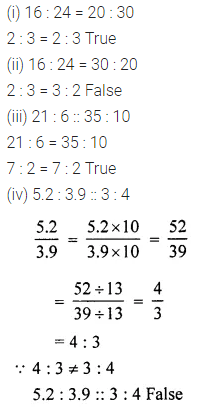Question 3.
Find which of the following are in proportion:
(i) 12, 16, 6, 8
(ii) 2, 3, 4, 5
(iii) 18, 10, 9, 5
(iv) 18, 9, 10, 5
Solution: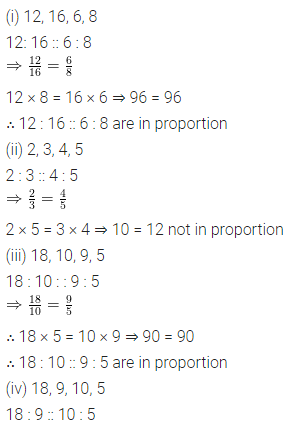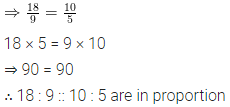Question 4.
Are the following statements true?
(i) 39 kg : 36 kg = 26 men : 24 men
(ii) 45 km : 60 km = 12 hours : 15 hours
(iii) 40 people : 200 people = ₹1000 : ₹5000
(iv) 7.5 litres: 15 litres = 15 children: 30 children
Solution:Question 5.
Determine if the following ratios form a proportion. Also, write the middle terms and extreme terms when the ratios form a proportion. .
(i) 25 cm : 1 m and ₹40 : ₹ 160
(ii) 39 litres : 65 litres and 6 bottles : 10 bottles
(iii) 2 kg : 80 kg and 30 sec : 5 minutes
(iv) 200 g : 2.5 kg and ₹4 : ₹50
Solution: